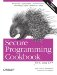# Computing the Distance Between Two Vectors

Problem

You want to find the Euclidean distance between two vectors.

Solution

The Euclidean distance between two vectors is defined as the square root of the sum of squares of differences between corresponding elements. This can be computed as shown in Example 11-22.

Example 11-22. Finding the distance between two vectors

```#include
#include

using namespace std;

template
double vectorDistance(Iter_T first, Iter_T last, Iter2_T first2) {
double ret = 0.0;
while (first != last) {
double dist = (*first++) - (*first2++);
ret += dist * dist;
}
return ret > 0.0 ? sqrt(ret) : 0.0;
}

int main( ) {
int v1[] = { 1, 5 };
int v2[] = { 4, 9 };
cout << "distance between vectors (1,5) and (4,9) is ";
cout << vectorDistance(v1, v1 + 2, v2) << endl;
}```

The program in Example 11-22 produces the following output:

`distance between vectors (1,5) and (4,9) is 5`

Discussion

Example 11-22 is a straightforward recipe that shows how to write a simple generic function in the style of the STL. To compute the vector distances, I could have instead used the inner_product function I chose not to use a functor, because it was more complex than was strictly needed. Example 11-23 shows how you can compute vector distance using a functor and the inner_product function from the header.

Example 11-23. Computing the distance between vectors using inner_product

```#include
#include
#include
#include

using namespace std;

template
struct DiffSquared {
Value_T operator( )(Value_T x, Value_T y) const {
return (x - y) * (x - y);
}
};

template
double vectorDistance(Iter_T first, Iter_T last, Iter2_T first2) {
double ret = inner_product(first, last, first2, 0.0L,
plus( ), DiffSquared( ));
return ret > 0.0 ? sqrt(ret) : 0.0;
}

int main( ) {
int v1[] = { 1, 5 };
int v2[] = { 4, 9 };
cout << "distance between vectors (1,5) and (4,9) is ";
cout << vectorDistance(v1, v1 + 2, v2) << endl;
}```

Because an implementation of inner_product( ) may contain special optimizations for your platform and compiler, I prefer to use it where possible.Secure Programming Cookbook for C and C++: Recipes for Cryptography, Authentication, Input Validation & More
ISBN: 0596003943
EAN: 2147483647
Year: 2006
Pages: 241